Introduction to Statistical Modeling with SAS/STAT Software
 Statistical Models

### Deterministic and Stochastic Models

Purely mathematical models, in which the relationships between inputs and outputs are captured entirely in deterministic fashion, can be important theoretical tools but are impractical for describing observational, experimental, or survey data. For such phenomena, researchers usually allow the model to draw on stochastic as well as deterministic elements. When the uncertainty of realizations leads to the inclusion of random components, the resulting models are called stochastic models. A statistical model, finally, is a stochastic model that contains parameters, which are unknown constants that need to be estimated based on assumptions about the model and the observed data.

There are many reasons why statistical models are preferred over deterministic models. For example:

• Randomness is often introduced into a system in order to achieve a certain balance or representativeness. For example, random assignment of treatments to experimental units allows unbiased inferences about treatment effects. As another example, selecting individuals for a survey sample by random mechanisms ensures a representative sample.

• Even if a deterministic model can be formulated for the phenomenon under study, a stochastic model can provide a more parsimonious and more easily comprehended description. For example, it is possible in principle to capture the result of a coin toss with a deterministic model, taking into account the properties of the coin, the method of tossing, conditions of the medium through which the coin travels and of the surface on which it lands, and so on. A very complex model is required to describe the simple outcome—heads or tails. Alternatively, you can describe the outcome quite simply as the result of a stochastic process, a Bernoulli variable that results in heads with a certain probability.

• It is often sufficient to describe the average behavior of a process, rather than each particular realization. For example, a regression model might be developed to relate plant growth to nutrient availability. The explicit aim of the model might be to describe how the average growth changes with nutrient availability, not to predict the growth of an individual plant. The support for the notion of averaging in a model lies in the nature of expected values, describing typical behavior in the presence of randomness. This, in turn, requires that the model contain stochastic components.

The defining characteristic of statistical models is their dependence on parameters and the incorporation of stochastic terms. The properties of the model and the properties of quantities derived from it must be studied in a long-run, average sense through expectations, variances, and covariances. The fact that the parameters of the model must be estimated from the data introduces a stochastic element in applying a statistical model: because the model is not deterministic but includes randomness, parameters and related quantities derived from the model are likewise random. The properties of parameter estimators can often be described only in an asymptotic sense, imagining that some aspect of the data increases without bound (for example, the number of observations or the number of groups).

The process of estimating the parameters in a statistical model based on your data is called fitting the model. For many classes of statistical models there are a number of procedures in SAS/STAT software that can perform the fitting. In many cases, different procedures solve identical estimation problems—that is, their parameter estimates are identical. In some cases, the same model parameters are estimated by different statistical principles, such as least squares versus maximum likelihood estimation. Parameter estimates obtained by different methods typically have different statistical properties—distribution, variance, bias, and so on. The choice between competing estimation principles is often made on the basis of properties of the estimators. Distinguishing properties might include (but are not necessarily limited to) computational ease, interprative ease, bias, variance, mean squared error, and consistency.

### Model-Based and Design-Based Randomness

A statistical model is a description of the data-generating mechanism, not a description of the specific data to which it is applied. The aim of a model is to capture those aspects of a phenomenon that are relevant to inquiry and to explain how the data could have come about as a realization of a random experiment. These relevant aspects might include the genesis of the randomness and the stochastic effects in the phenomenon under study. Different schools of thought can lead to different model formulations, different analytic strategies, and different results. Coarsely, you can distinguish between a viewpoint of innate randomness and one of induced randomness. This distinction leads to model-based and design-based inference approaches.

In a design-based inference framework, the random variation in the observed data is induced by random selection or random assignment. Consider the case of a survey sample from a finite population of size
; suppose that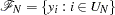denotes the finite set of possible values and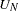is the index set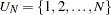. Then a sample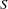, a subset of, is selected by probability rules. The realization of the random experiment is the selection of a particular set; the associated values selected from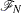are considered fixed. If properties of a design-based sampling estimator are evaluated, such as bias, variance, and mean squared error, they are evaluated with respect to the distribution induced by the sampling mechanism.

Design-based approaches also play an important role in the analysis of data from controlled experiments by randomization tests. Suppose thattreatments are to be assigned to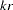homogeneous experimental units. If you formsets ofunits with equal probability, and you assign theth treatment to theth set, a completely randomized experimental design (CRD) results. A design-based view treats the potential response of a particular treatment for a particular experimental unit as a constant. The stochastic nature of the error-control design is induced by randomly selecting one of the potential responses.

Statistical models are often used in the design-based framework. In a survey sample the model is used to motivate the choice of the finite population parameters and their sample-based estimators. In an experimental design, an assumption of additivity of the contributions from treatments, experimental units, observational errors, and experimental errors leads to a linear statistical model. The approach to statistical inference where statistical models are used to construct estimators and their properties are evaluated with respect to the distribution induced by the sample selection mechanism is known as model-assisted inference (Särndal, Swensson, and Wretman 1992).

In a purely model-based framework, the only source of random variation for inference comes from the unknown variation in the responses. Finite population values are thought of as a realization of a superpopulation model that describes random variables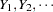. The observed values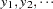are realizations of these random variables. A model-based framework does not imply that there is only one source of random variation in the data. For example, mixed models might contain random terms that represent selection of effects from hierarchical (super-) populations at different granularity. The analysis takes into account the hierarchical structure of the random variation, but it continues to be model based.

A design-based approach is implicit in SAS/STAT procedures whose name commences with SURVEY, such as the SURVEYFREQ, SURVEYMEANS, SURVEYREG, and SURVEYLOGISTIC procedures. Inferential approaches are model based in other SAS/STAT procedures. For more information about analyzing survey data with SAS/STAT software, see Chapter 14, Introduction to Survey Sampling and Analysis Procedures.

### Model Specification

If the model is accepted as a description of the data-generating mechanism, then its parameters are estimated using the data at hand. Once the parameter estimates are available, you can apply the model to answer questions of interest about the study population. In other words, the model becomes the lens through which you view the problem itself, in order to ask and answer questions of interest. For example, you might use the estimated model to derive new predictions or forecasts, to test hypotheses, to derive confidence intervals, and so on.

Obviously, the model must be "correct" to the extent that it sufficiently describes the data-generating mechanism. Model selection, diagnosis, and discrimination are important steps in the model-building process. This is typically an iterative process, starting with an initial model and refining it. The first important step is thus to formulate your knowledge about the data-generating process and to express the real observed phenomenon in terms of a statistical model. A statistical model describes the distributional properties of one or more variables, the response variables. The extent of the required distributional specification depends on the model, estimation technique, and inferential goals. This description often takes the simple form of a model with additive error structure:

response = mean + error

In mathematical notation this simple model equation becomes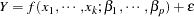In this equation
is the response variable, often also called the dependent variable or the outcome variable. The terms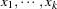denote the values ofregressor variables, often termed the covariates or the "independent" variables. The terms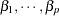denote parameters of the model, unknown constants that are to be estimated. The termdenotes the random disturbance of the model; it is also called the residual term or the error term of the model.

In this simple model formulation, stochastic properties are usually associated only with theterm. The covariatesare usually known values, not subject to random variation. Even if the covariates are measured with error, so that their values are in principle random, they are considered fixed in most models fit by SAS/STAT software. In other words, stochastic properties under the model are derived conditional on thes. Ifis the only stochastic term in the model, and if the errors have a mean of zero, then the function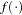is the mean function of the statistical model. More formally,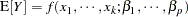where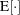denotes the expectation operator.

In many applications, a simple model formulation is inadequate. It might be necessary to specify not only the stochastic properties of a single error term, but also how model errors associated with different observations relate to each other. A simple additive error model is typically inappropriate to describe the data-generating mechanism if the errors do not have zero mean or if the variance of observations depends on their means. For example, ifis a Bernoulli random variable that takes on the valuesandonly, a regression model with additive error is not meaningful. Models for such data require more elaborate formulations involving probability distributions.Previous Page | Next Page | Top of Page